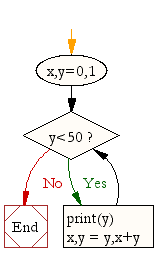## ~ OOP,Classes,Python,Tkinter ...recreating PONG

### Code and Challenge

Copy and paste the code below. Run it! Read the task and see if you can do it before moving on to the next challenge

```"""
Experiment with the movement of the bat - how can you fine tune it to your specifications/requirements
"""

from tkinter import *
import random
import time

class Ball:
def __init__(self,canvas,color):
self.canvas=canvas
self.id=canvas.create_oval(30,30,50,50,fill=color)
self.canvas.move(self.id,100,200)
starting_position=[-3,-2,-1,1,2,3]
random.shuffle(starting_position)
self.x = starting_position
self.y = -3
self.canvas_height=self.canvas.winfo_height()
self.canvas_width=self.canvas.winfo_width()

def draw(self):
self.canvas.move(self.id,self.x,self.y)
pos=self.canvas.coords(self.id)
if pos <=0:
self.y=6
if pos >=self.canvas_height:
self.y=-6
if pos <=0:
self.x=6
if pos>=self.canvas_width:
self.x=-6

#===PONG BAT CLASS====
class Pongbat():
def __init__(self,canvas,color):
self.canvas=canvas
self.id=canvas.create_rectangle(0,0,100,10,fill=color)
self.canvas.move(self.id,200,300)
self.x=0 #add the x object variable here in the __init__ function of our bat class
self.canvas_width=self.canvas.winfo_width() #create a variable for the canvas width, as we did with the ball
#Binding the methods/functions leftturn and rightturn below to the correct keys
self.canvas.bind_all('',self.left_turn)
self.canvas.bind_all('',self.right_turn)

def draw(self): #add to the draw function (similar to the ball function's method)
self.canvas.move(self.id,self.x,0) #move the bat in the direction of the x variable
pos=self.canvas.coords(self.id)
if pos<=0: #if the left x coordinate is less than or equal to 0, then we don't want the bat to bounce, we want it to STOP
self.x=0
if pos>=self.canvas_width: #same situation here, when the right x coordinate is greater than or equal to the width, then stop!
self.x=0

#===ADD FUNCTIONS /METHODS FOR CHANGING DIRECTION BETWEEN LEFT AND RIGHT
#Note: We use binding to bind these functions to the correct key (e.g. left or right) in the__init__function
def left_turn(self,evt):
self.x=-10 #change this variable to increase or decrease the speed of the pong bat!

def right_turn(self,evt):
self.x=10

def main():
tk=Tk()
tk.title("My 21st Century Pong Game")
tk.resizable(0,0)
tk.wm_attributes("-topmost",1)
canvas=Canvas(tk,bg="white",width=500,height=400,bd=0,highlightthickness=0)
canvas.pack()
tk.update()

ball1=Ball(canvas,'green')
bat1=Pongbat(canvas,'red')
while 1:
tk.update()
ball1.draw()
bat1.draw()
time.sleep(0.02)
main()

#note - an ERROR may be caused as Python is complaining about breaking out of the while loop when the window is closed - you can ignore that for now (or a fix is provied in the solutions section for those who are interested"""

```

Systems Life Cycle (in a nutshell): Analyse - Design - Create - Test - Evaluate. Designing something or writing out some pseudocode before you actually write code is always a good idea! Get in to the habit of doing so! You can draw your flowchart here and screenshot it.

A sample flow chart (design) for this particular challenge could look like:Each challenge section below provides an online drawing tool where you can dynamically create flowcharts. Screenshot them into your presentation for submission.